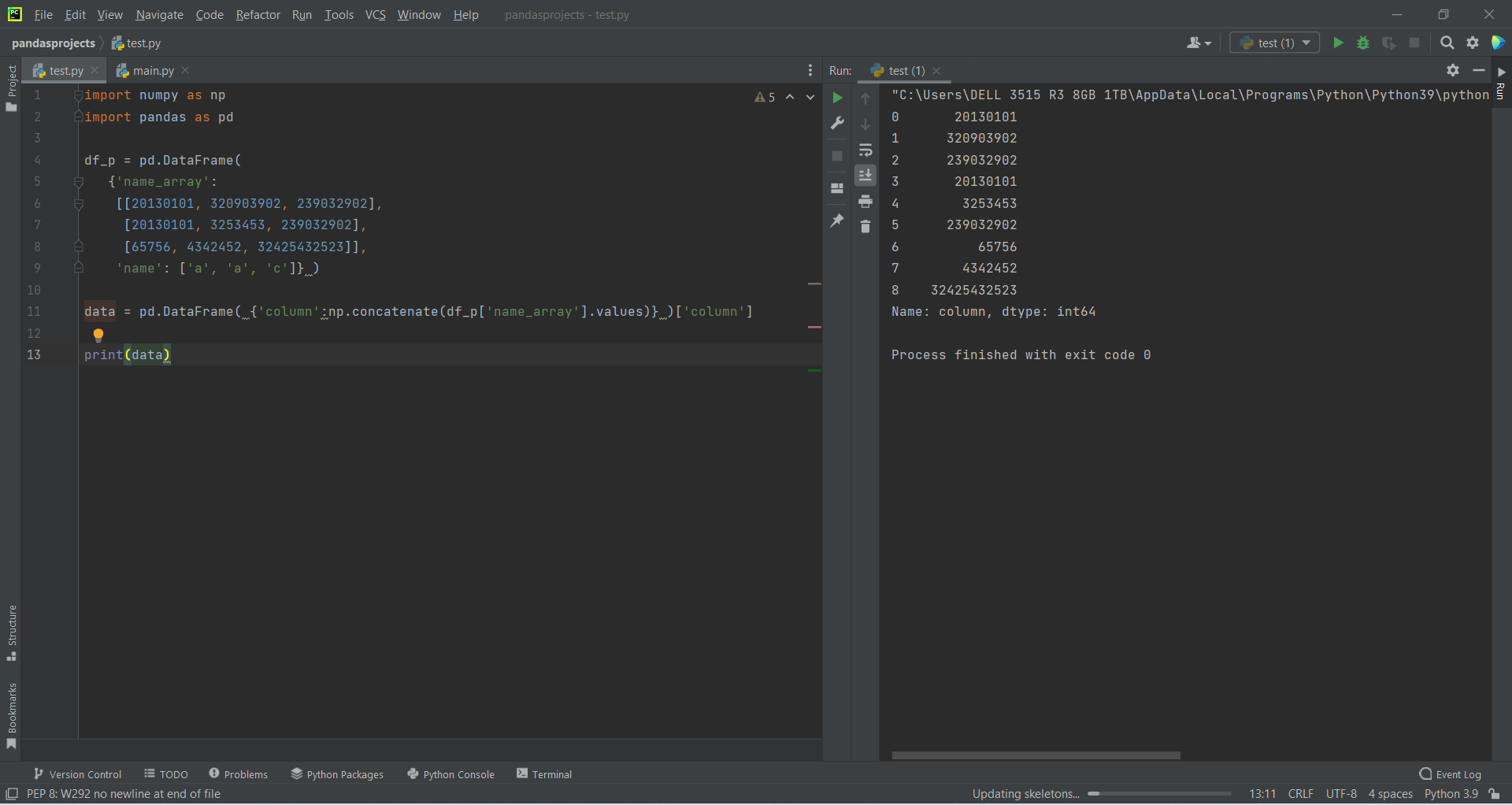# Flatten Array in Pandas Dataframeby Abdul Rawoof A RUpdated: Feb 28, 2023Solution Kit

In Python, a process of reducing the dimensionality of an array is known as a Flattening array, and it is the process of lowering the number of array dimensions to a lower number.

Index.flatten(order='C') returns a copy of the array collapsed into one dimension and the parameters: order: {'C', 'F', 'A'}, optional. Whether to flatten in C (row-major), Fortran (column-major) order, or preserve the C/Fortran ordering from a and the first method to flatten the pandas dataframe is through the NumPy python package. There is a function in NumPy that is numpy in the Python package.flatten() function that performs this task. Priorly, we must convert the dataframe to numpy using the to_numpy() method and then apply the flatten() method. By using ndarray.flatten() function, and then we can flatten a matrix to one dimension in Python, order:'C' means to flatten in row-major, and 'F' means to flatten in column-major.

The use of flatten function is that FLATTEN is a table function in Python that takes a VARIANT, ARRAY, or OBJECT column and produces a lateral view, that is, an inline view that includes correlation referring to other tables that precede it in the FROM clause and it can convert semi-structured data to a relational representation.

Here is an example of how to implement a flattened array in Pandas DataFrame:Fig : Preview of the output that you will get on running this code from your IDE.

### Code

In this solution we're using Pandas and NumPy libraries.

### Instructions

Follow the steps carefully to get the output easily.

2. Copy the snippet using the 'copy' and paste it in your IDE.
3. Add required dependencies and import them in Python file.
4. Run the file to generate the output.

I hope you found this useful. I have added the link to dependent libraries, version information in the following sections.

I found this code snippet by searching for 'how to flatten array in pandas dataframe' in kandi. You can try any such use case!

### Environment Tested

I tested this solution in the following versions. Be mindful of changes when working with other versions.

1. The solution is created in PyCharm 2021.3.
2. The solution is tested on Python 3.9.7.
3. Pandas version-v1.5.2.

Using this solution, we are able to flatten array to pandas data frame with simple steps. This process also facilities an easy way to use, hassle-free method to create a hands-on working version of code which would help us to flatten array to pandas data frame.

### Dependent Libraries

pandasby pandas-dev

Python38689Version:v2.0.2Flexible and powerful data analysis / manipulation library for Python, providing labeled data structures similar to R data.frame objects, statistical functions, and much more

Support
Quality
Security
Reuse

pandasby pandas-dev

Python38689Version:v2.0.2License: Permissive (BSD-3-Clause)

Flexible and powerful data analysis / manipulation library for Python, providing labeled data structures similar to R data.frame objects, statistical functions, and much more
Support
Quality
Security
Reuse

numpyby numpy

Python23755Version:v1.25.0rc1The fundamental package for scientific computing with Python.

Support
Quality
Security
Reuse

numpyby numpy

Python23755Version:v1.25.0rc1License: Permissive (BSD-3-Clause)

The fundamental package for scientific computing with Python.
Support
Quality
Security